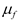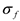Spitzer Documentation & Tools
IRS Instrument Handbook

### 5.7.2             RIDGE

The RIDGE module finds the peak in the spatial profile that was produced by the PROFILE module. In other words, it defines the spectral trace of the source to be extracted. The peak is represented as a percent value (from 0.0 to 100.0) of the slit length. By default the module computes this peak position. Alternatively, the peak position can be forced to have a specified value, set by the input parameter Cut Percentage, also denoted c.  The module takes as input the file profile.tbl, and the two calibration files wavsamp.tbl and psf_fov.tbl.

The search for the peak position (if Cut Percentage is not given) is performed within plus or minus Percent Range (a parameter, also denoted as g) of the position expected based on the selected field-of-view. In the pipeline, Percent Range = 25% for SL and LL, meaning that, for low-resolution data, the search for a peak is performed within a spatial window of width 50% of the slit length, centered on the commanded nod position. For SH and LH, instead of the parameter g, the parameters Percent Low = 25% and Percent High = 75% (also denoted glo and ghi, respectively) are used in the pipeline. They supersede g and as a result RIDGE searches for a peak in a window of width 50% of the slit length, centered on the slit.

The search algorithm is as follows:

Ę  Read in the data in profile.tbl (as a function of percent length along the slit): y0

Ę  Calculate a linear fit to y0, and subtract it from the data to obtain yh

Ę  Perform median filtering of this flattened yh data, using a number of points Median_Pts to get yf. In the pipeline, the parameter Median_Pts (also denoted as m) has value 5.

Ę  Calculate the new mean,and sigma,, for the median-filtered data yf

Ę  Find the peak value mh in the unfiltered, flattened data, yh

Ę  The profile has a candidate peak ifEquation 5.18

In the pipeline, the parameter Num_Sigma (also denoted s) has value 5.0.

The final cut percentage P is then set to the position of the candidate peak found above. But if no candidate peak is found, P is set to the expected position of the field-of-view as defined by the DFRAC column of the psf_fov.tbl file.

Once P is determined, the RIDGE module computes the ridge line, defined to be the locus of the cut percentage P at each of the pseudo-rectangles (measured from the leftmost edge of each). It outputs the ASCII table ridge.tbl, containing the ridge line, in the form of IRS array coordinates (x,y) of the peak for each order and each wavelength. In actuality, two sets of coordinates (x,y) are output at each wavelength; they are the left and right edges of the nominal extraction window used by EXTRACT, centered at P.